# 题目描述（中等难度）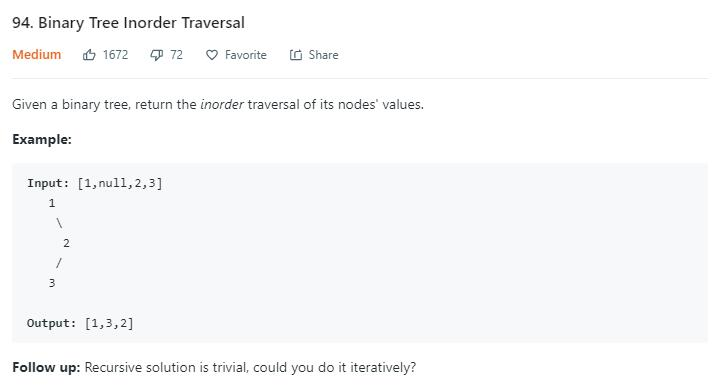# 解法一 递归

public List<Integer> inorderTraversal(TreeNode root) {
List<Integer> ans = new ArrayList<>();
getAns(root, ans);
return ans;
}

private void getAns(TreeNode node, List<Integer> ans) {
if (node == null) {
return;
}
getAns(node.left, ans);
getAns(node.right, ans);
}


# 解法二 栈

//当前节点为空，出栈
if (node == null) {
return;
}
//当前节点不为空
getAns(node.left, ans);  //压栈
getAns(node.right, ans); //压栈
//左右子树遍历完，出栈



1
/   \
2     3
/ \   /
4   5 6

push   push   push   pop     pop    push     pop     pop
|   |  |   |  |_4_|  |   |   |   |  |   |    |   |   |   |
|   |  |_2_|  |_2_|  |_2_|   |   |  |_5_|    |   |   |   |
|_1_|  |_1_|  |_1_|  |_1_|   |_1_|  |_1_|    |_1_|   |   |
[]                        [4 2]           [4 2 5] [4 2 5 1]
push   push   pop          pop
|   |  |   |  |   |        |   |
|   |  |_6_|  |   |        |   |
|_3_|  |_3_|  |_3_|        |   |
[4 2 5 1 6]  [4 2 5 1 6 3]


public List<Integer> inorderTraversal(TreeNode root) {
List<Integer> ans = new ArrayList<>();
Stack<TreeNode> stack = new Stack<>();
TreeNode cur = root;
while (cur != null || !stack.isEmpty()) {
//节点不为空一直压栈
while (cur != null) {
stack.push(cur);
cur = cur.left; //考虑左子树
}
//节点为空，就出栈
cur = stack.pop();
//当前值加入
//考虑右子树
cur = cur.right;
}
return ans;
}


# 解法三 Morris Traversal

1、cur.left 为 null，保存 cur 的值，更新 cur = cur.right

2、cur.left 不为 null，找到 cur.left 这颗子树最右边的节点记做 last

2.1 last.right 为 null，那么将 last.right = cur，更新 cur = cur.left

2.2 last.right 不为 null，说明之前已经访问过，第二次来到这里，表明当前子树遍历完成，保存 cur 的值，更新 cur = cur.right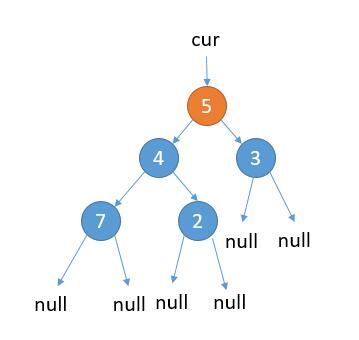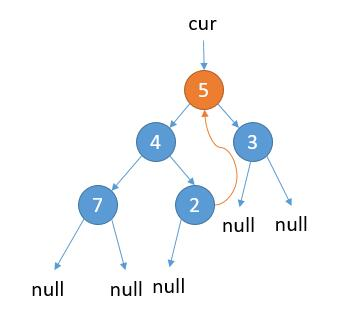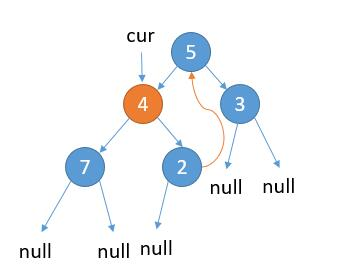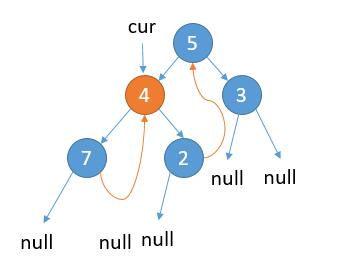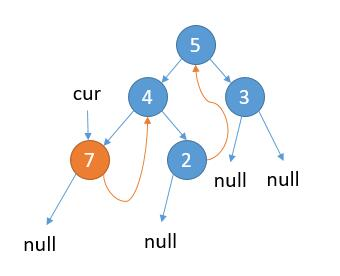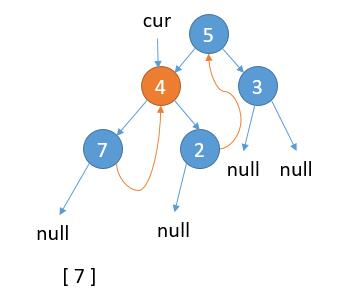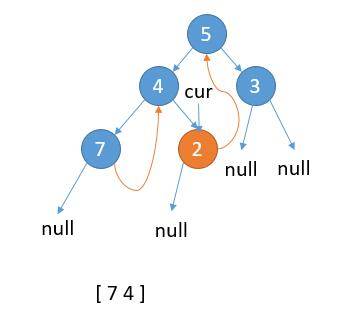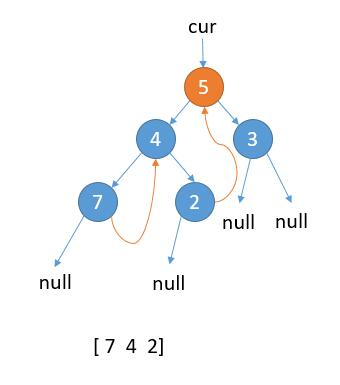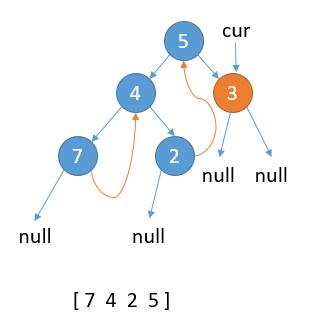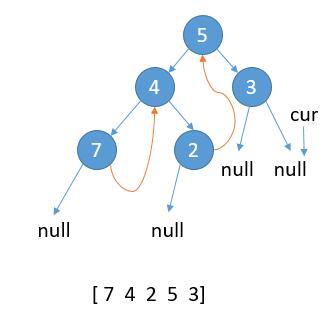cur 指向 null，结束遍历。

1、cur.left 为 null，保存 cur 的值，更新 cur = cur.right

2、cur.left 不为 null，找到 cur.left 这颗子树最右边的节点记做 last

2.1 last.right 为 null，那么将 last.right = cur，更新 cur = cur.left

2.2 last.right 不为 null，说明之前已经访问过，第二次来到这里，表明当前子树遍历完成，保存 cur 的值，更新 cur = cur.right

public List<Integer> inorderTraversal(TreeNode root) {
List<Integer> ans = new ArrayList<>();
TreeNode cur = root;
while (cur != null) {
//情况 1
if (cur.left == null) {
cur = cur.right;
} else {
//找左子树最右边的节点
TreeNode pre = cur.left;
while (pre.right != null && pre.right != cur) {
pre = pre.right;
}
//情况 2.1
if (pre.right == null) {
pre.right = cur;
cur = cur.left;
}
//情况 2.2
if (pre.right == cur) {
pre.right = null; //这里可以恢复为 null
cur = cur.right;
}
}
}
return ans;
}